# RD Sharma Solutions for Class 9 Maths Chapter 17 Construction

## RD Sharma Solutions Class 9 Maths Chapter 17 – Free PDF Download

RD Sharma Solutions for Class 9 Maths Chapter 17 – Constructions is given in detail for students to understand the covered concepts in a more comprehensive manner. All the questions are solved with the help of figures for easy and better understanding. The RD Sharma Solutions are well structured by expert faculty for a better understanding of the concepts, which helps students prepare for their exams more effectively. The solutions are presented in simple language based on the current CBSE syllabus updated for the 2021-22 exams.

In geometry, constructions refer to the drawing of angles, lines, and various other shapes accurately using only a compass and a ruler. These constructions are considered as a pure form of geometric constructions since no other measuring devices like protractors are used. This method of construction was used by Euclid for various basic constructions including bisecting a line, drawing different angles, constructing shapes like triangles, etc. All questions of Chapter 17 Constructions are given according to the exercises in the RD Sharma Solutions Class 9 Maths textbook.

## Download PDF of RD Sharma Solutions for Class 9 Maths Chapter 17 Construction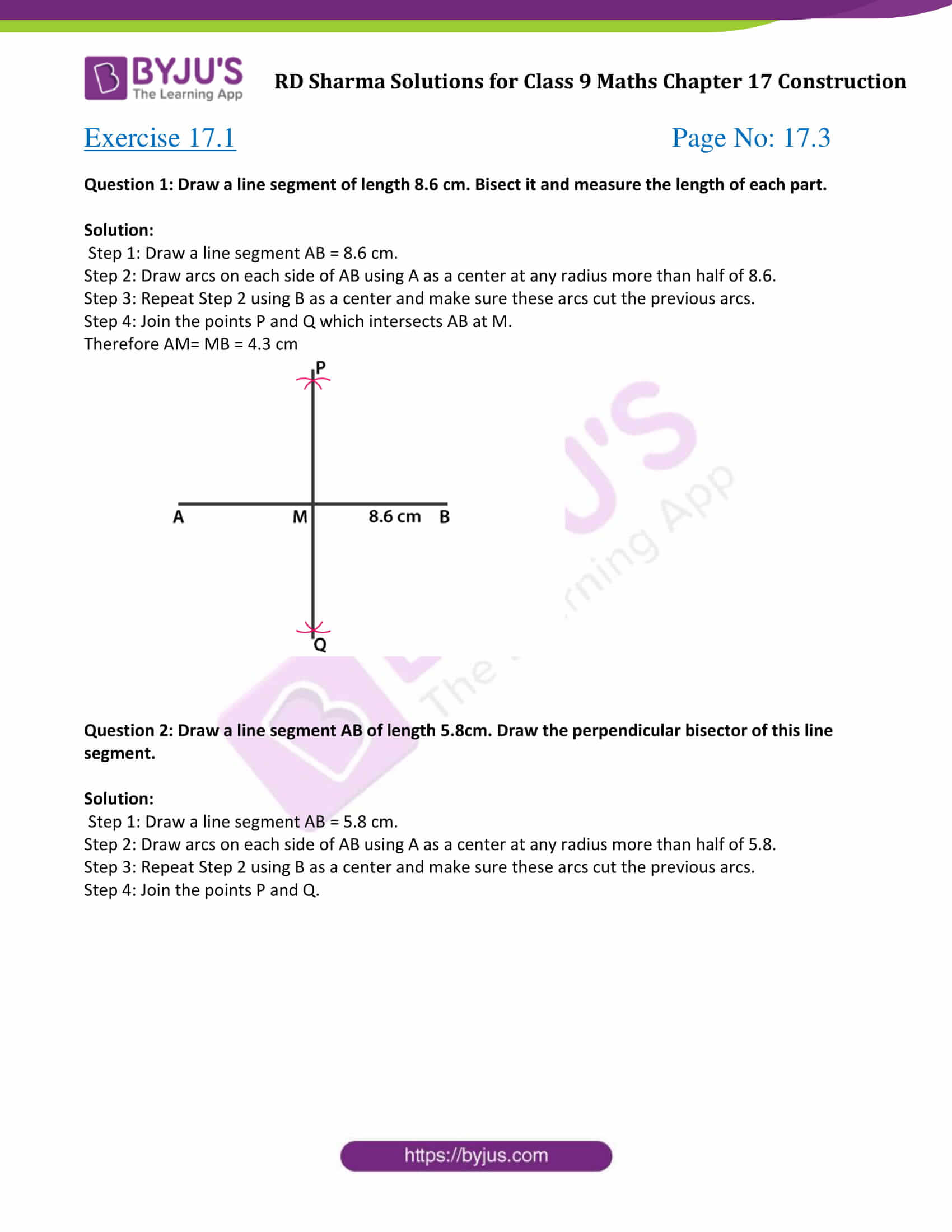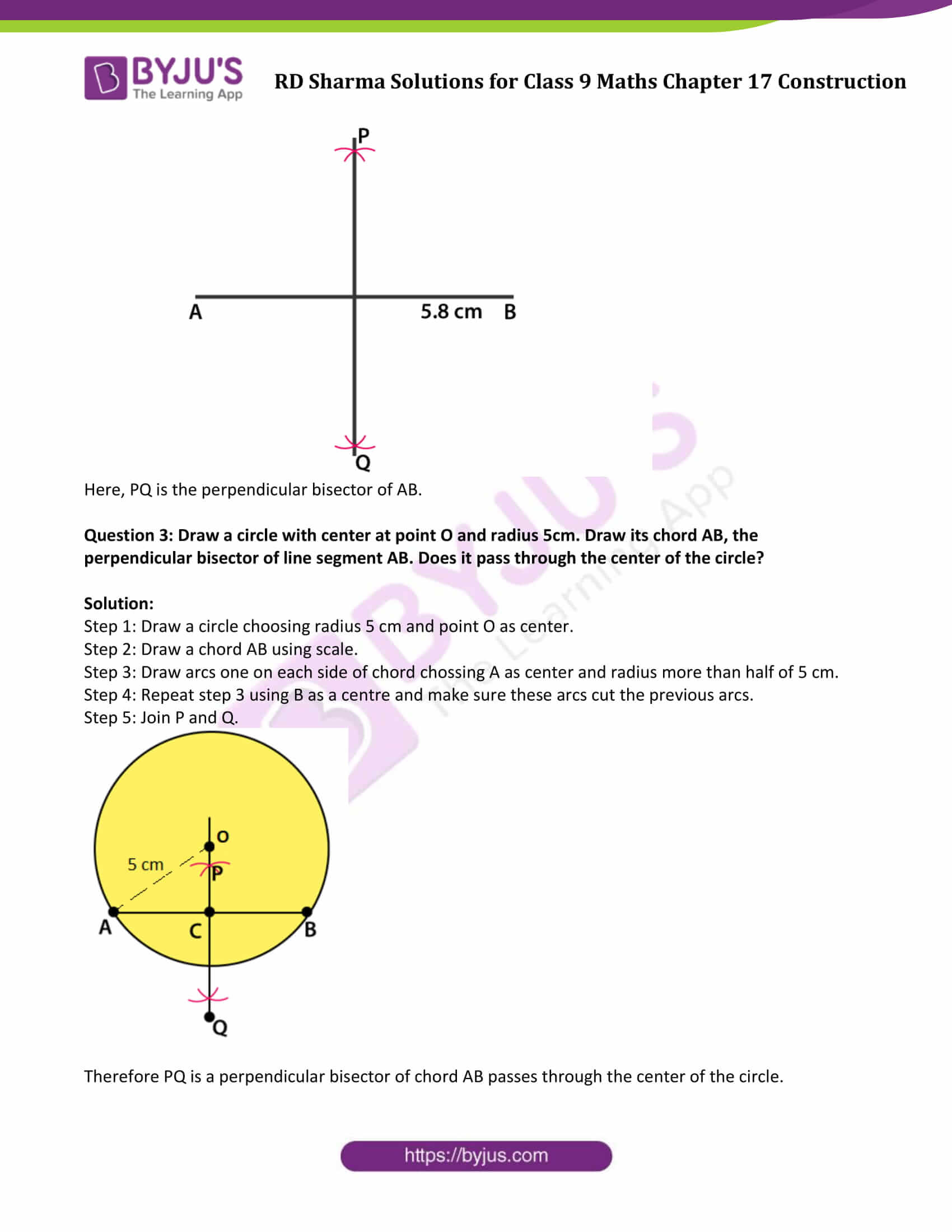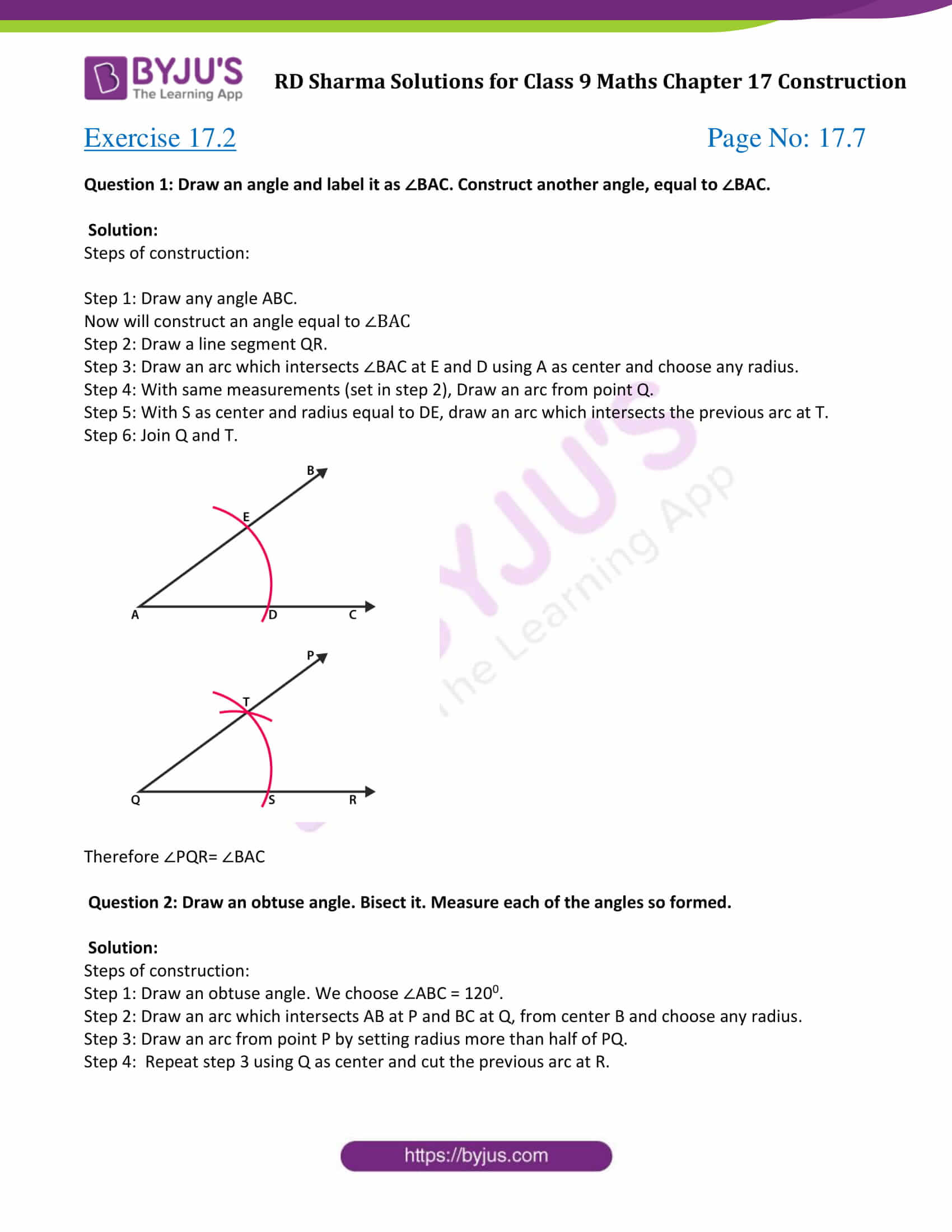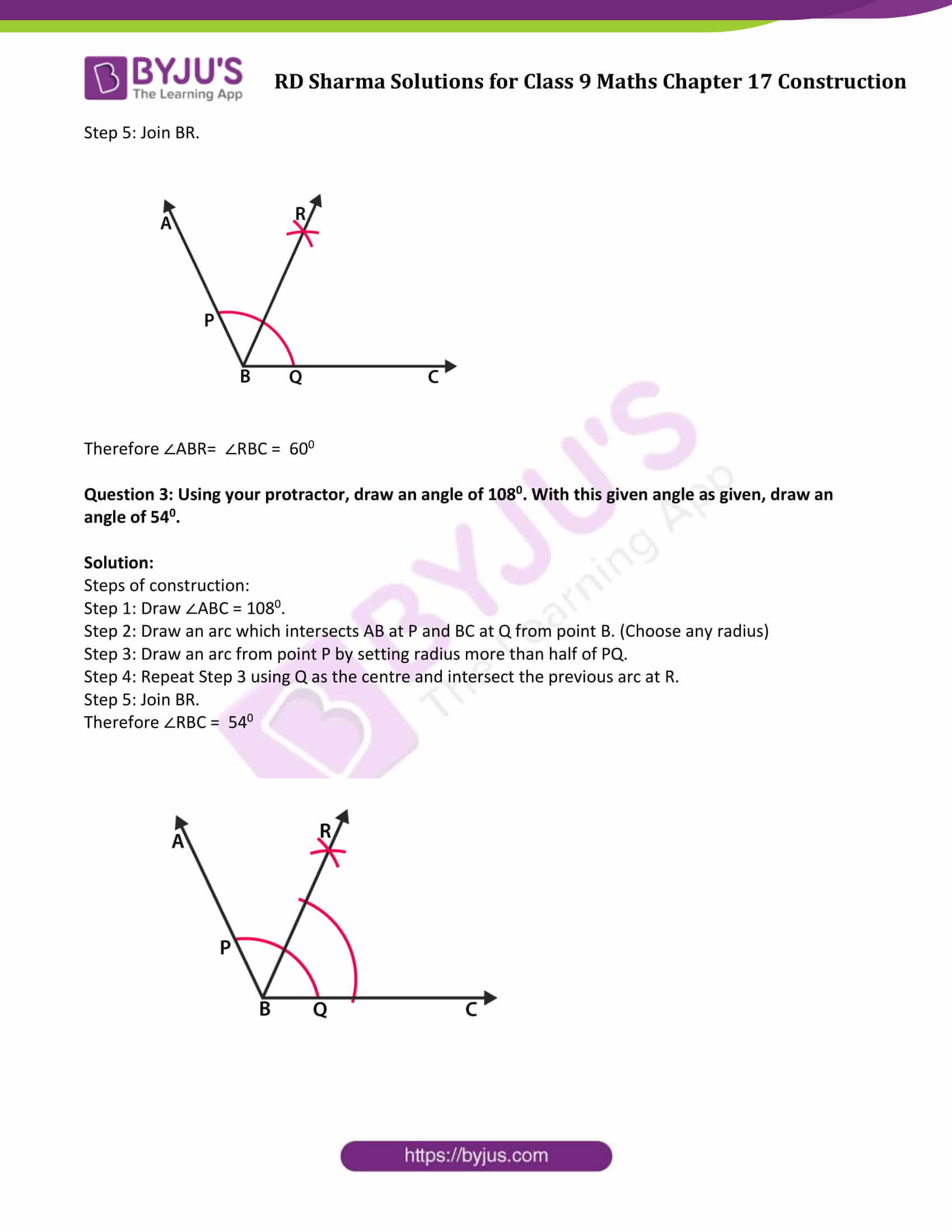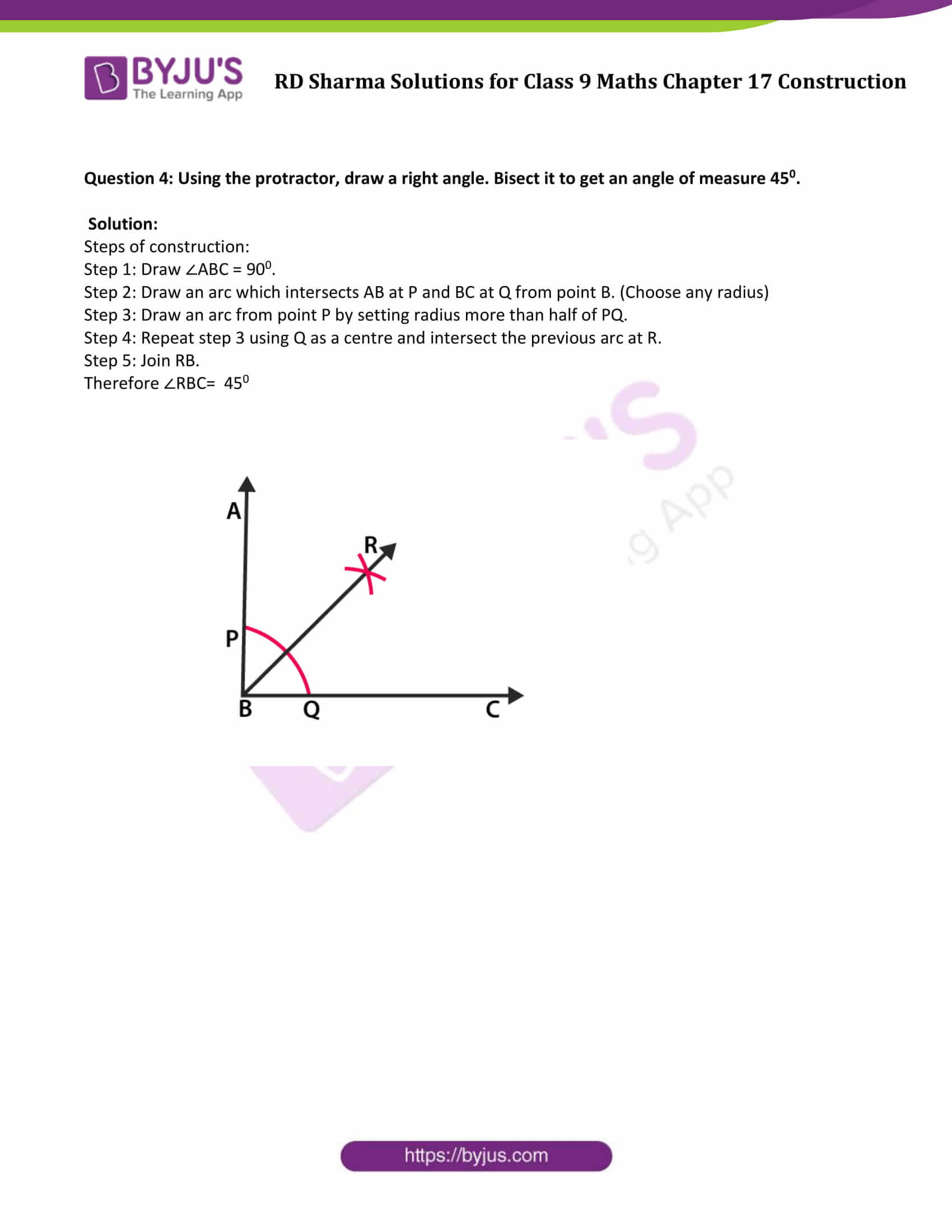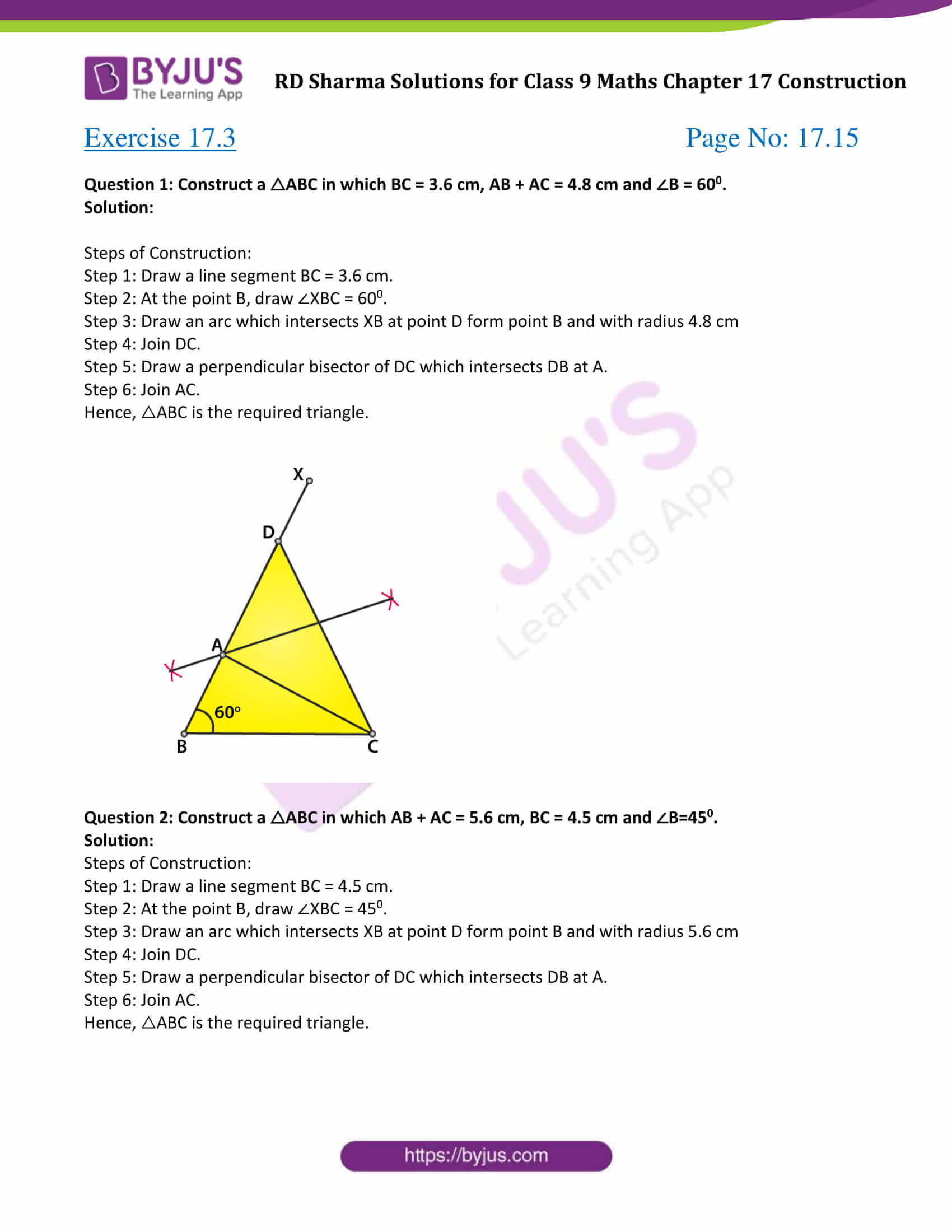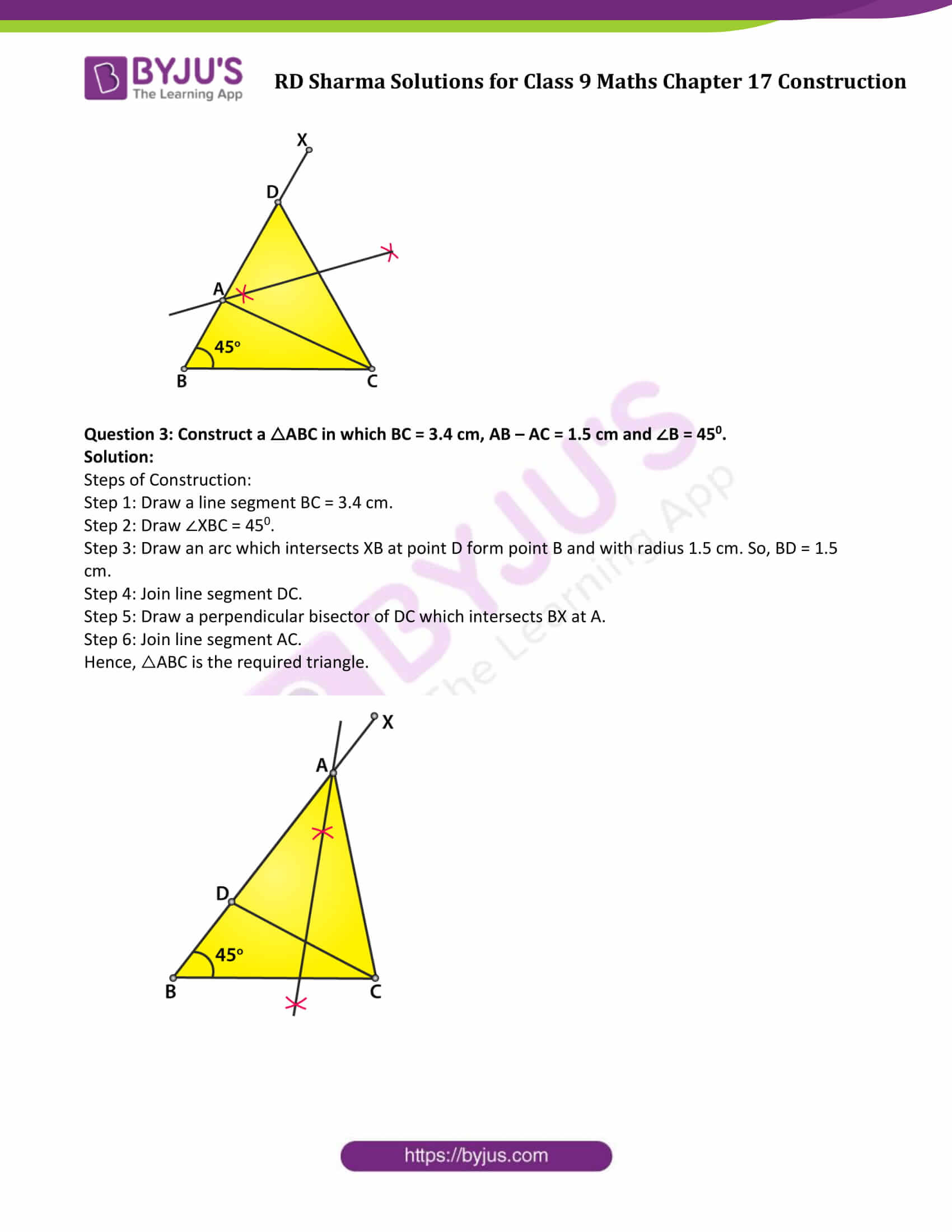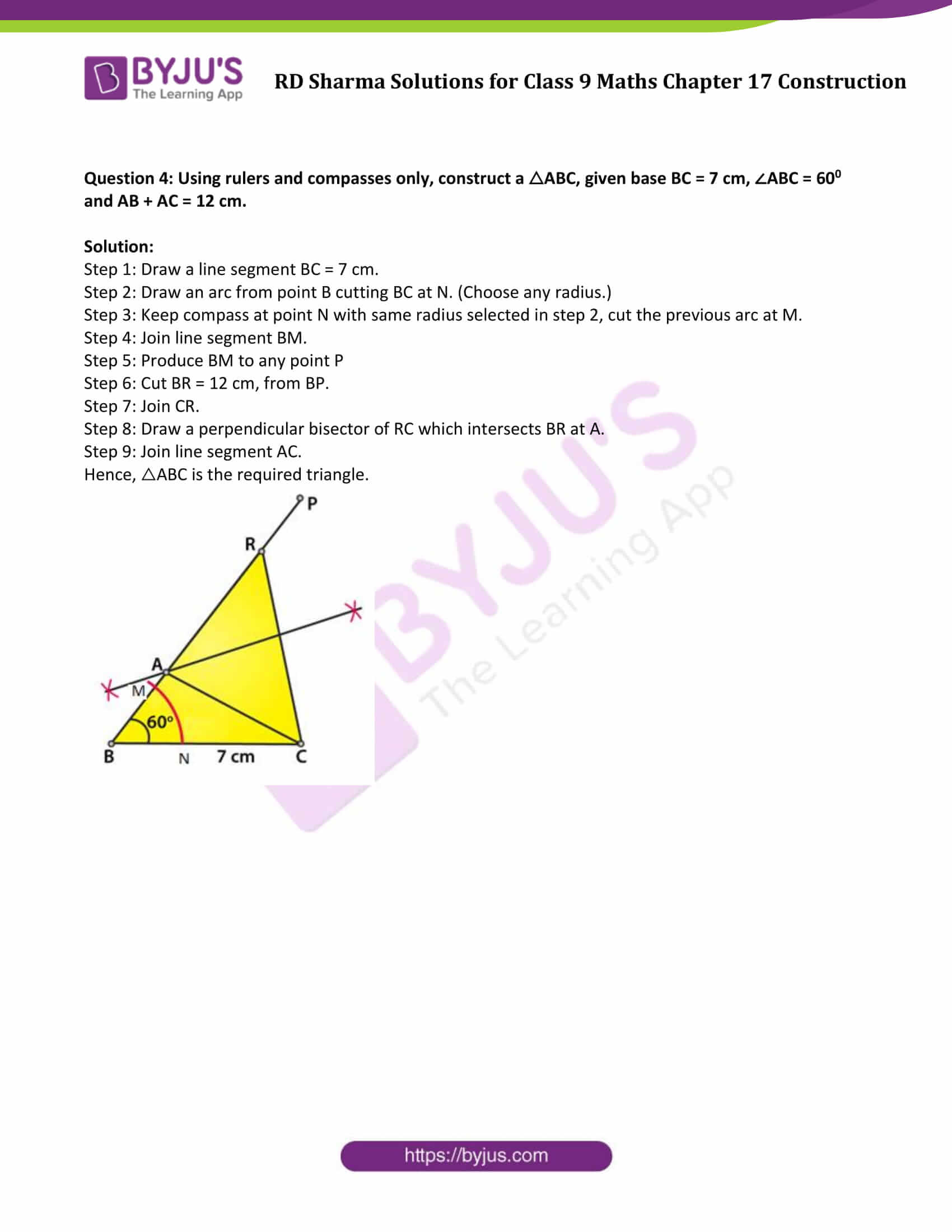### Exercise 17.1 Page No: 17.3

Question 1: Draw a line segment of length 8.6 cm. Bisect it and measure the length of each part.

Solution:

Step 1: Draw a line segment AB = 8.6 cm.

Step 2: Draw arcs on each side of AB using A as a center at any radius more than half of 8.6.

Step 3: Repeat Step 2 using B as a center and make sure these arcs cut the previous arcs.

Step 4: Join the points P and Q which intersects AB at M.

Therefore AM= MB = 4.3 cm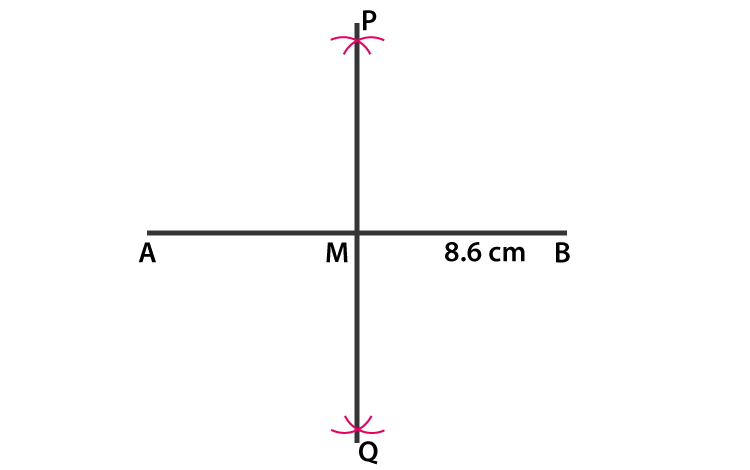Question 2: Draw a line segment AB of length 5.8cm. Draw the perpendicular bisector of this line segment.

Solution:

Step 1: Draw a line segment AB = 5.8 cm.

Step 2: Draw arcs on each side of AB using A as a center at any radius more than half of 5.8.

Step 3: Repeat Step 2 using B as a center and make sure these arcs cut the previous arcs.

Step 4: Join the points P and Q.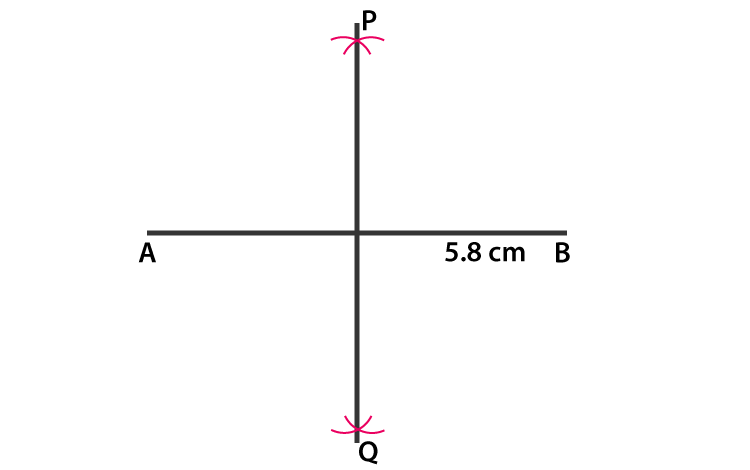Here, PQ is the perpendicular bisector of AB.

Question 3: Draw a circle with center at point O and radius 5cm. Draw its chord AB, the perpendicular bisector of line segment AB. Does it pass through the center of the circle?

Solution:

Step 1: Draw a circle choosing radius 5 cm and point O as center.

Step 2: Draw a chord AB using scale.

Step 3: Draw arcs one on each side of chord chossing A as center and radius more than half of 5 cm.

Step 4: Repeat step 3 using B as a centre and make sure these arcs cut the previous arcs.

Step 5: Join P and Q.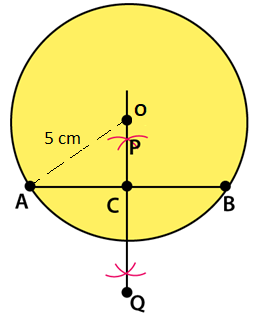Therefore PQ is a perpendicular bisector of chord AB passes through the center of the circle.

### Exercise 17.2 Page No: 17.7

Question 1: Draw an angle and label it as ∠BAC. Construct another angle, equal to ∠BAC.

Solution:

Steps of construction:

Step 1: Draw any angle ABC.

Now will construct an angle equal to ∠BAC

Step 2: Draw a line segment QR.

Step 3: Draw an arc which intersects ∠BAC at E and D using A as center and choose any radius.

Step 4: With same measurements (set in step 2), Draw an arc from point Q.

Step 5: With S as center and radius equal to DE, draw an arc which intersects the previous arc at T.

Step 6: Join Q and T.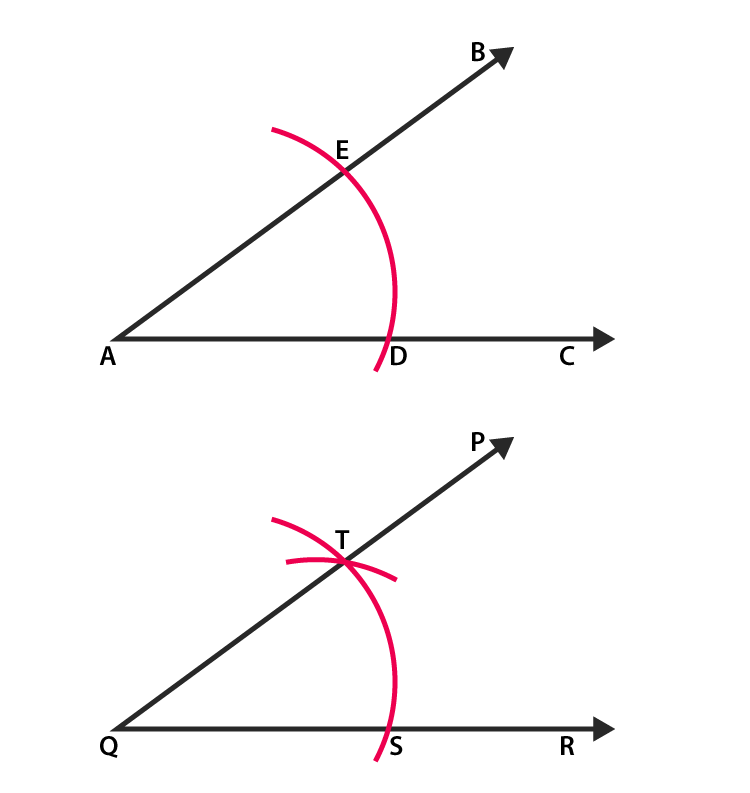Therefore ∠PQR= ∠BAC

Question 2: Draw an obtuse angle. Bisect it. Measure each of the angles so formed.

Solution:

Steps of construction:

Step 1: Draw an obtuse angle. We choose ∠ABC = 1200.

Step 2: Draw an arc which intersects AB at P and BC at Q, from center B and choose any radius.

Step 3: Draw an arc from point P by setting radius more than half of PQ.

Step 4: Repeat step 3 using Q as center and cut the previous arc at R.

Step 5: Join BR.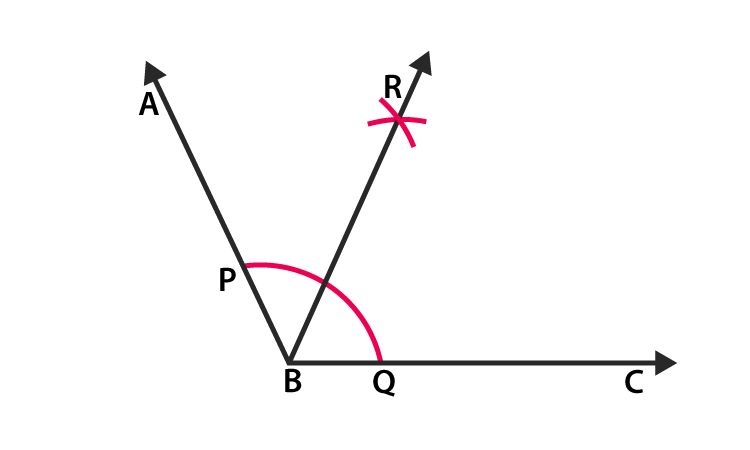Therefore ∠ABR= ∠RBC = 600

Question 3: Using your protractor, draw an angle of 1080. With this given angle as given, draw an angle of 540.

Solution:

Steps of construction:

Step 1: Draw ∠ABC = 1080.

Step 2: Draw an arc which intersects AB at P and BC at Q from point B. (Choose any radius)

Step 3: Draw an arc from point P by setting radius more than half of PQ.

Step 4: Repeat Step 3 using Q as the centre and intersect the previous arc at R.

Step 5: Join BR.

Therefore ∠RBC = 540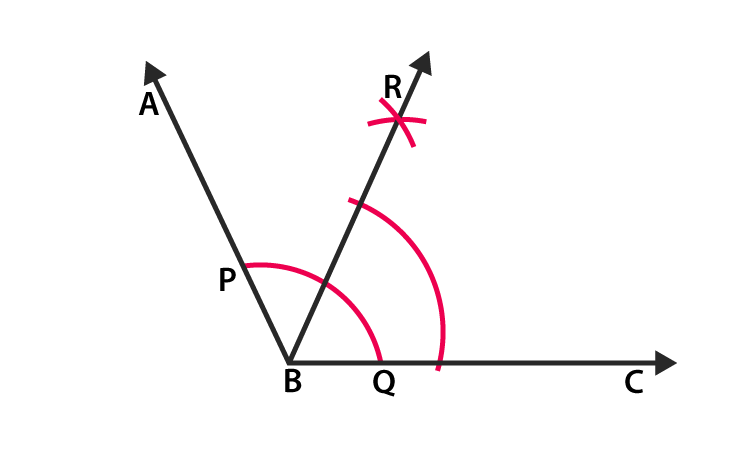Question 4: Using the protractor, draw a right angle. Bisect it to get an angle of measure 450.

Solution:

Steps of construction:

Step 1: Draw ∠ABC = 900.

Step 2: Draw an arc which intersects AB at P and BC at Q from point B. (Choose any radius)

Step 3: Draw an arc from point P by setting radius more than half of PQ.

Step 4: Repeat step 3 using Q as a centre and intersect the previous arc at R.

Step 5: Join RB.

Therefore ∠RBC= 450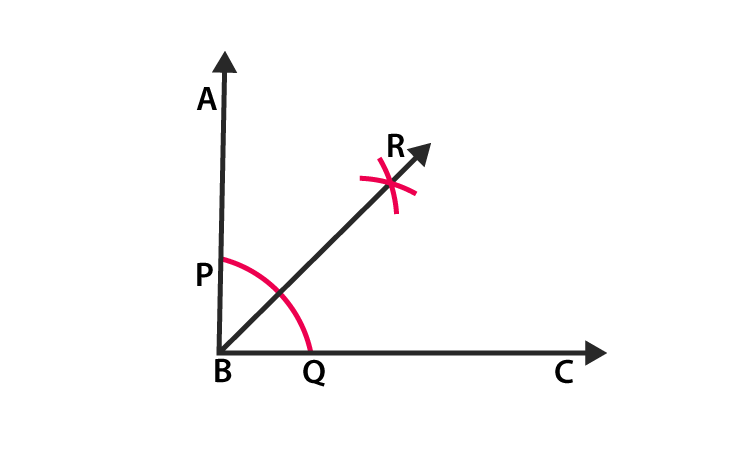### Exercise 17.3 Page No: 17.15

Question 1: Construct a △ABC in which BC = 3.6 cm, AB + AC = 4.8 cm and ∠B = 600.

Solution:

Steps of Construction:

Step 1: Draw a line segment BC = 3.6 cm.

Step 2: At the point B, draw ∠XBC = 600.

Step 3: Draw an arc which intersects XB at point D form point B and with radius 4.8 cm

Step 4: Join DC.

Step 5: Draw a perpendicular bisector of DC which intersects DB at A.

Step 6: Join AC.

Hence, △ABC is the required triangle.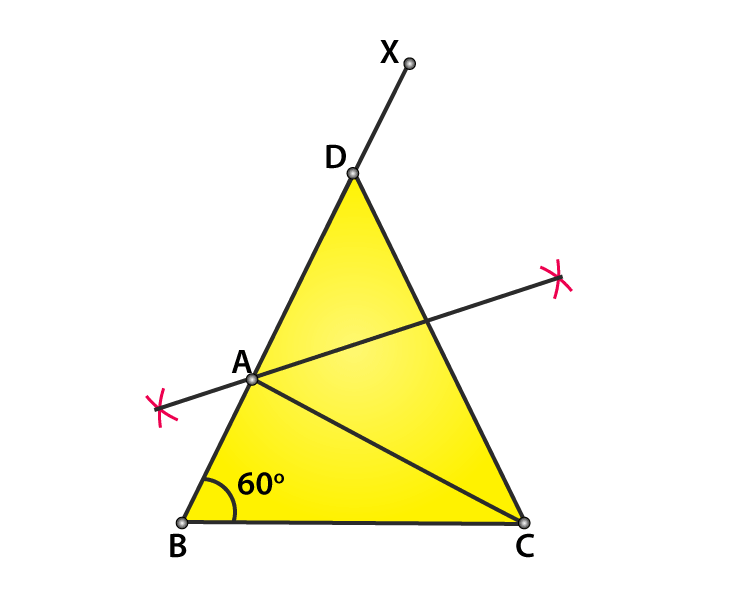Question 2: Construct a △ABC in which AB + AC = 5.6 cm, BC = 4.5 cm and ∠B=450.

Solution:

Steps of Construction:

Step 1: Draw a line segment BC = 4.5 cm.

Step 2: At the point B, draw ∠XBC = 450.

Step 3: Draw an arc which intersects XB at point D form point B and with radius 5.6 cm

Step 4: Join DC.

Step 5: Draw a perpendicular bisector of DC which intersects DB at A.

Step 6: Join AC.

Hence, △ABC is the required triangle.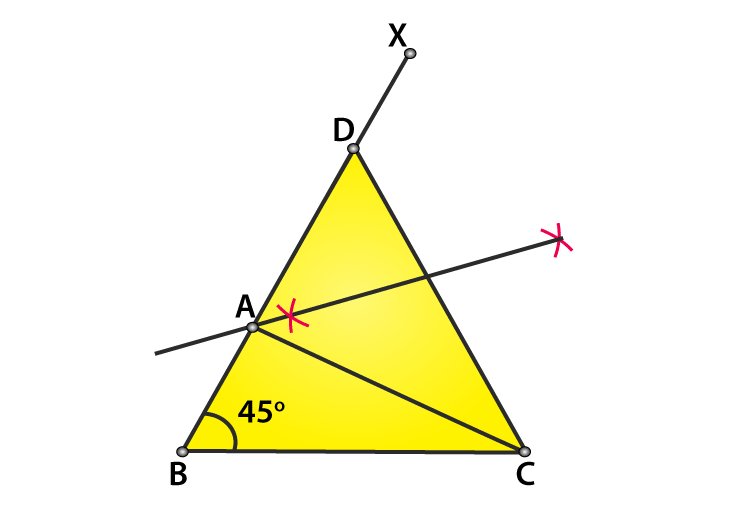Question 3: Construct a △ABC in which BC = 3.4 cm, AB – AC = 1.5 cm and ∠B = 450.

Solution:

Steps of Construction:

Step 1: Draw a line segment BC = 3.4 cm.

Step 2: Draw ∠XBC = 450.

Step 3: Draw an arc which intersects XB at point D form point B and with radius 1.5 cm. So, BD = 1.5 cm.

Step 4: Join line segment DC.

Step 5: Draw a perpendicular bisector of DC which intersects BX at A.

Step 6: Join line segment AC.

Hence, △ABC is the required triangle.Question 4: Using rulers and compasses only, construct a △ABC, given base BC = 7 cm, ∠ABC = 600 and AB + AC = 12 cm.

Solution:

Step 1: Draw a line segment BC = 7 cm.

Step 2: Draw an arc from point B cutting BC at N. (Choose any radius.)

Step 3: Keep compass at point N with same radius selected in step 2, cut the previous arc at M.

Step 4: Join line segment BM.

Step 5: Produce BM to any point P

Step 6: Cut BR = 12 cm, from BP.

Step 7: Join CR.

Step 8: Draw a perpendicular bisector of RC which intersects BR at A.

Step 9: Join line segment AC.

Hence, △ABC is the required triangle.### RD Sharma Solutions for Class 9 Maths Chapter 17 Construction

In the 17th Chapter of Class 9 RD Sharma Solutions students will study important concepts listed below:

• Construction Introduction
• Construction of Bisector of a Line Segment
• Construction of the Bisector of a Given Angle
• Constructions of Triangles and many more

## Frequently Asked Questions on RD Sharma Solutions for Class 9 Maths Chapter 17

### How does RD Sharma Solutions for Class 9 Maths Chapter 17 help students?

RD Sharma Solutions for Class 9 Maths Chapter 17 are used by students to get a proper grasp of all the concepts of the subjects and also to lay the foundation for their career or further higher studies. BYJU’S experts formulate these questions in an easy and understandable manner that helps students solve problems in the most efficient possible ways. We hope these solutions will help CBSE Class 9 students, to build a strong foundation of the basics and secure excellent marks in their final exam.

### Why should we follow RD Sharma Solutions for Class 9 Maths Chapter 17?

RD Sharma Solutions for Class 9 Maths Chapter 17 is the correct learning strategy that is devised to help them master the concepts. Revising the textbook problems referring to these solutions will help students crack any problems asked in the exams. The accurate answers developed by faculty help to boost the problem solving skills along with logical reasoning among students. These solutions are the most popular study materials used by the students to excel in CBSE board exams. Practising these solutions helps the students to top the final exams and obtain proficiency in the subject.

### Where to download RD Sharma Solutions for Class 9 Maths Chapter 17?

RD Sharma Solutions for Class 9 Maths Chapter 17 can be downloaded by the students in offline mode or can be referred online from the BYJU’S website. These solutions are formulated by BYJU’S expert faculty, in an efficient manner in accordance with the current CBSE syllabus.